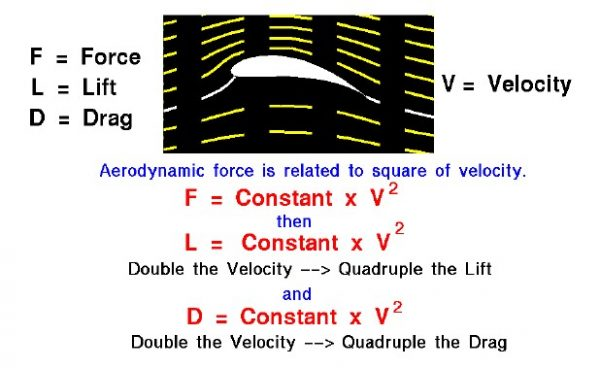# Effects of Velocity on Lift Interactive## Fluid Momentum

Lift is created by deflecting a flow of air and drag is generated on a body in a wide variety of ways. From Newton’s second law of motion, the aerodynamic forces on the body (lift and drag) are directly related to the change in momentum of the fluid with time. The fluid momentum is equal to the mass times the velocity of the fluid. Since the air moves, defining the mass gets a little tricky. Modern aerodynamicists relate the effect of mass on lift and drag to the air density. At the time of the Wright brothers, the effect of mass was included in the Smeaton pressure coefficient. In both systems, the lift and drag depend on the square of the velocity.

## Relative Velocity

The velocity used in the lift and drag equations is the relative velocity between an object and the flow. Since the aerodynamic force depends on the square of the velocity, doubling the velocity will quadruple the lift and drag.

You can investigate the dependence of lift on velocity using this JavaScript program.

Please note: the simulation below is best viewed on a desktop computer. It may take a few minutes for the simulation to load.

## Instructions

You can change the value of the velocity by using the slider below the airfoil graphic, or by backspacing, typing in your value, and hitting “Return” inside the input box next to the slider. You can perform the calculations in either English or metric units by using the drop menu labeled “Select Units”. The red dot on the graph shows the current set of conditions.

As an experiment, set the velocity to 50 mph and note the amount of lift. Now double the velocity to 100 mph. What is the value of the lift? How does it compare to the previous measurement?

Provide feedback